# The CALIS Procedure

### Example 29.29 Fitting the RAM and EQS Models by the COSAN Modeling Language

Subsections:

The COSAN modeling language in PROC CALIS enables you to specify the direct or implied mean and covariance structures for the data in terms of matrix formulas. It is a very general modeling language, and all other modeling languages in PROC CALIS are special cases of the COSAN modeling language. This example shows how you can apply the COSAN modeling language to situations where you might usually use the "easier" modeling languages. Therefore, the purpose of this example is not to recommend the use of the COSAN modeling specification to the specific application. Rather, through its connections with other more well-known model types, this example intends to help you understand the basics of the COSAN modeling language.

In Example 29.17, you fit a path model to the Wheaton data (Wheaton et al. 1977) by using the PATH modeling language. The mathematical basis of the PATH modeling language is the RAM model. In Example 29.23, you use the RAM and LINEQS statements to specify the same path model. In all these different types of specifications, you specify the functional relationships of the variables and the variance and covariance parameters in the model. PROC CALIS then generates the implied covariance structures for analysis internally. The COSAN modeling language is quite different. In the COSAN statement, you specify the covariance structures directly as a matrix formula. This example shows how you can do that in two different ways. One specification emulates the RAM model (McDonald 1978, 1980) covariance structures and the other emulates the EQS model (Bentler 1995) covariance structures.

#### Emulating the RAM model by the COSAN Modeling Language

In the RAM model, you specify all information regarding the path effects or coefficients (that is, single-headed arrows in the path diagram) in the so-called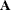(_A_) matrix. You specify all the information regarding the variances and covariances (that is, the double-headed arrows in the path diagram) in the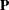(_P_) matrix. See the section The RAM Model for more details about the mathematical model for RAM. Once you define these two matrices, the implied covariance structures for the observed variables are derived by the formula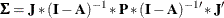whereis an identity matrix and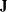is a selection matrix that contains 0 or 1 as its elements for selecting the covariance structures elements for the observed variables.

For example, in the RAM model specification in Example 29.23, you essentially use the following RAM model specification:

proc calis nobs=932 data=Wheaton primat nose;
ram
var =  Anomie67     /* 1 */
Powerless67  /* 2 */
Anomie71     /* 3 */
Powerless71  /* 4 */
Education    /* 5 */
SEI          /* 6 */
Alien67      /* 7 */
Alien71      /* 8 */
SES,         /* 9 */
_A_    1   7   1.0,
_A_    2   7   0.833,
_A_    3   8   1.0,
_A_    4   8   0.833,
_A_    5   9   1.0,
_A_    6   9   lambda,
_A_    7   9   gamma1,
_A_    8   9   gamma2,
_A_    8   7   beta,
_P_    1   1   theta1,
_P_    2   2   theta2,
_P_    3   3   theta1,
_P_    4   4   theta2,
_P_    5   5   theta3,
_P_    6   6   theta4,
_P_    7   7   psi1,
_P_    8   8   psi2,
_P_    9   9   phi,
_P_    1   3   theta5,
_P_    2   4   theta5;
run;


In the RAM statement, you specify all the parameters in the _A_ and _P_ matrices, and PROC CALIS generates the corresponding covariance structures for analysis. However, with the COSAN modeling language, in addition to the parameter in the model matrices, you need to supply the matrix formula for the covariance structures, as shown in the preceding formula for
.

Before discussing how you can specify the COSAN model that corresponds to this RAM model specification, it is useful to look at the initial model matrices that are generated by the preceding RAM model specification. To do this, you use the PRIMAT option in the PROC CALIS statement.

Output 29.29.1 and Output 29.29.2 show the initial _A_ and _P_ matrices, respectively, for the RAM model.

Output 29.29.1: Initial _A_ Matrix of the RAM Model

Initial RAM _A_ Matrix
Anomie67 Powerless67 Anomie71 Powerless71 Education SEI Alien67 Alien71 SES
Anomie67
 0
 0
 0
 0
 0
 0
 1.0000
 0
 0
Powerless67
 0
 0
 0
 0
 0
 0
 0.8330
 0
 0
Anomie71
 0
 0
 0
 0
 0
 0
 0
 1.0000
 0
Powerless71
 0
 0
 0
 0
 0
 0
 0
 0.8330
 0
Education
 0
 0
 0
 0
 0
 0
 0
 0
 1.0000
SEI
 0
 0
 0
 0
 0
 0
 0
 0
 . [lambda]
Alien67
 0
 0
 0
 0
 0
 0
 0
 0
 . [gamma1]
Alien71
 0
 0
 0
 0
 0
 0
 . [beta]
 0
 . [gamma2]
SES
 0
 0
 0
 0
 0
 0
 0
 0
 0

Output 29.29.2: Initial _P_ Matrix of the RAM Model

Initial RAM _P_ Matrix
Anomie67 Powerless67 Anomie71 Powerless71 Education SEI Alien67 Alien71 SES
Anomie67
 . [theta1]
 0
 . [theta5]
 0
 0
 0
 0
 0
 0
Powerless67
 0
 . [theta2]
 0
 . [theta5]
 0
 0
 0
 0
 0
Anomie71
 . [theta5]
 0
 . [theta1]
 0
 0
 0
 0
 0
 0
Powerless71
 0
 . [theta5]
 0
 . [theta2]
 0
 0
 0
 0
 0
Education
 0
 0
 0
 0
 . [theta3]
 0
 0
 0
 0
SEI
 0
 0
 0
 0
 0
 . [theta4]
 0
 0
 0
Alien67
 0
 0
 0
 0
 0
 0
 . [psi1]
 0
 0
Alien71
 0
 0
 0
 0
 0
 0
 0
 . [psi2]
 0
SES
 0
 0
 0
 0
 0
 0
 0
 0
 . [phi]

Essentially, to specify the same model by the COSAN modeling language, you need to provide the same information in these two initial model matrices and the covariance structure formula forin the COSAN model specification, which is shown in the following statements:

proc calis data=Wheaton nobs=932 nose;
cosan
var= Anomie67 Powerless67 Anomie71 Powerless71 Education SEI,
J(9, IDE) * A(9, GEN, IMI) * P(9, SYM);
matrix  A
[1 2 8   , 7] = 1.0  0.833  beta,
[3 4     , 8] = 1.0  0.833 ,
[5 6 7 8 , 9] = 1.   lambda  gamma1  gamma2;
matrix P
[1,1] = theta1-theta2 theta1-theta4 ,
[7,7] = psi1 psi2 phi,
[3,1] = theta5 ,
[4,2] = theta5 ;
vnames
J = [Anomie67 Powerless67 Anomie71 Powerless71
Education SEI Alien67 Alien71 SES],
A = J,
P = A;
run;


In the PROC CALIS statement, you provide the data set in the DATA= option and the number of observations in the NOBS= option. You use the NOSE option to turn off the computation of the standard error estimates.

In the VAR= option of the COSAN statement, you provide the list of observed variables for the analysis. You do not specify the latent variables in the VAR= option in the COSAN statement as you do in the VAR= option in the RAM statement. Then, you specify the formula for the covariance structures for the set of variables in the VAR= list. Because the covariance structure formula is symmetric, you only need to specify "half" of it. That is, the specification J(9,IDE)*A(9,GEN,IMI)*P(9,SYM) in the COSAN statement automatically expands to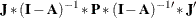which is the required covariance structures. The arguments in the matrices represent the number of columns, the matrix type, and the transformation type (optional), respectively. For example, the notation A(9, GEN, IMI) means that matrixhas nine columns and it is a general (GEN) rectangular or square matrix. You do not specify the number of rows for matrixexplicitly, but PROC CALIS can deduce that because matrixfollows matrixin the multiplication. To make matrix multiplication conformable, the number of rows for matrixmust be the same as the number of columns for matrix, which is nine. The IMI notation means the identity-minus-inverse transformation, which results in putting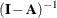in the expression. Matrixin the covariance structure formula is a 99 symmetric matrix. It does not have any transformation in the formula. Matrixin the covariance structure formula is a so-called generalized identity matrix (IDE), which has six rows and nine columns. Basically, you use this matrix to select the observed variables in the covariance structure formula. The exact form of this matrix will become clear when the PROC CALIS output is shown.

Next, you use two MATRIX statements to specify the parameters in the model matricesand, for RAM model matrices _A_ and _P_, respectively. For example, in the first entry of the MATRIX statement for thematrix, you specify the elements [1,7], [2,7], and [8,7] by 1.0, 0.833, and beta, respectively. The first two elements are fixed constants, while the last one is a free parameter named beta. Similarly, you specify all the fixed or free parameters in matrix, which reflects the same pattern you specify for the _A_ matrix of the RAM model, as shown in Output 29.29.1.

For thematrix, you specify the parameters in the same fashion. Becauseis defined as a symmetric matrix, you need to specify only the lower triangular elements. In the first entry of the MATRIX statement for thematrix, you specify the [1,1] element, but the trailing parameter list has six parameters. The [1,1] notation here is interpreted as the starting location of the matrix. It proceeds to [2,2], [3,3], [4,4] and so on. The length of the trailing parameter list determines the number of elements being specified. Therefore, the last parameter in this entry is for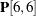, which is a free parameter theta4. Similarly, you define all other parameters in thematrix, which reflects the same pattern you specify for the _P_ matrix of the RAM model, as shown in Output 29.29.2.

In the VNAMES statement, you can specify the column variable names for the model matrices. You provide a set of nine variable names for the column of matrixin the pairs of brackets. The first six names are those of the observed variables in the COSAN model, while the last six names are for latent factors. How about the row variable names for matrix? Because matrixis the first matrix in the covariance structure formula, its row names are automatically the same as the names of the observed variables in the VAR= list of the COSAN statement. Next, you specify the column variable names of matrix. You equate that to matrix, meaning that the column variable names in matrixare the same those for matrix. How about the row variable names for matrix? Because matrixfollows matrixin the covariance structure formula, its row names are automatically same as the column names for matrix. Lastly, you define that the column names for matrixare the same as those for matrix.

Notice that column names serve only as labels. PROC CALIS does not know the identities of the row and column variables. For example, the first column of matrixis Anomie67, which is also a name for an observed variable in the COSAN model. Keeping other specifications intact, you could name this column by any other name without affecting the model estimation. It is recommended that you use sensible names that help you remember the identities of the row and column variables, such as this example shows.

Output 29.29.3 shows the modeling information and the observed variables in the COSAN model. PROC CALIS analyzed the covariance structures of the six observed variables listed in Output 29.29.3.

Output 29.29.3: Modeling Information of the COSAN Model for the Wheaton Data: RAM Emulation

Modeling Information
Maximum Likelihood Estimation
Data Set WORK.WHEATON
N Obs 932
Model Type COSAN
Analysis Covariances

Observed Variables (N = 6) in the Model
Anomie67 Powerless67 Anomie71 Powerless71 Education SEI

Output 29.29.4 shows the covariance structures and some properties of the model matrices. The covariance structure formula for Sigma is defined as required. You can also check the matrix properties in this output to see if they are what you intend them to be.

Output 29.29.4: The Covariance Structures and Model Matrices of the COSAN Model for the Wheaton Data: RAM Emulation

COSAN Model Structures
Sigma = J*inv(_I_-A)*P*(inv(_I_-A))*J

Summary of Model Matrices
Matrix N Row N Col Matrix Type
A 9 9 GEN: Square
J 6 9 IDE: (I || 0)
P 9 9 SYM: Symmetric

Output 29.29.4 shows thatis a 69 "identity" matrix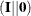. Essentially,is a selection matrix that contains either 0 or 1 as its elements. The role of matrixin the covariance structure formula is to extract first six rows and columns in the inner covariance structures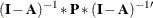(which is 99) to form the covariance structures only for the observed variables (which is 66). But how can this identity matrix have more columns (9) than rows (6)? In common mathematical notation, an identity matrix must always be a square matrix. However, for convenience in notation, PROC CALIS generalizes it to the IDE type. An IDE matrix that has the same numbers of columns and rows is a square identity matrix. If an IDE matrix has more columns than rows, it denotes an identity matrix concatenated (to the right) by a null matrix (that is, thenotation). If an IDE matrix has more rows than columns, it denotes an identity matrix appended (to the bottom) by a null matrix (that is, the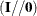notation). The generalized definition for the IDE matrix offers an efficient way to define selection matrix, such as thematrix shown in this example.

Output 29.29.5 shows the model fit chi-square of the COSAN model. This is the same model fit as in Output 29.17.7 of Example 29.17, as expected.

Output 29.29.5: Model Fit of the COSAN Model for the Wheaton Data: RAM Emulation

Fit Summary
Chi-Square 13.4851
Chi-Square DF 9
Pr > Chi-Square 0.1419

Output 29.29.6 shows the estimates in thematrix.

Output 29.29.6: Estimate of theMatrix by the COSAN Model Specification

Model Matrix A
(9 x 9 General Square Matrix)
Anomie67 Powerless67 Anomie71 Powerless71 Education SEI Alien67 Alien71 SES
Anomie67
 0
 0
 0
 0
 0
 0
 1.0000
 0
 0
Powerless67
 0
 0
 0
 0
 0
 0
 0.8330
 0
 0
Anomie71
 0
 0
 0
 0
 0
 0
 0
 1.0000
 0
Powerless71
 0
 0
 0
 0
 0
 0
 0
 0.8330
 0
Education
 0
 0
 0
 0
 0
 0
 0
 0
 1.0000
SEI
 0
 0
 0
 0
 0
 0
 0
 0
 5.3689 [lambda]
Alien67
 0
 0
 0
 0
 0
 0
 0
 0
 -0.6299 [gamma1]
Alien71
 0
 0
 0
 0
 0
 0
 0.5931 [beta]
 0
 -0.2409 [gamma2]
SES
 0
 0
 0
 0
 0
 0
 0
 0
 0

The estimates in Output 29.29.6 from the COSAN model specification are essentially the same as those from the RAM model specification, as shown in the matrix form in Output 29.29.7.

Output 29.29.7: Estimate of theMatrix by the RAM Model Specification

RAM _A_ Matrix
Anomie67 Powerless67 Anomie71 Powerless71 Education SEI Alien67 Alien71 SES
Anomie67
 0
 0
 0
 0
 0
 0
 1.0000
 0
 0
Powerless67
 0
 0
 0
 0
 0
 0
 0.8330
 0
 0
Anomie71
 0
 0
 0
 0
 0
 0
 0
 1.0000
 0
Powerless71
 0
 0
 0
 0
 0
 0
 0
 0.8330
 0
Education
 0
 0
 0
 0
 0
 0
 0
 0
 1.0000
SEI
 0
 0
 0
 0
 0
 0
 0
 0
 5.3688 [lambda]
Alien67
 0
 0
 0
 0
 0
 0
 0
 0
 -0.6299 [gamma1]
Alien71
 0
 0
 0
 0
 0
 0
 0.5931 [beta]
 0
 -0.2409 [gamma2]
SES
 0
 0
 0
 0
 0
 0
 0
 0
 0

Output 29.29.8 shows the estimates in thematrix.

Output 29.29.8: Estimate of theMatrix by the COSAN Model Specification

Model Matrix P
(9 x 9 Symmetric Matrix)
Anomie67 Powerless67 Anomie71 Powerless71 Education SEI Alien67 Alien71 SES
Anomie67
 3.6078 [theta1]
 0
 0.9058 [theta5]
 0
 0
 0
 0
 0
 0
Powerless67
 0
 3.5950 [theta2]
 0
 0.9058 [theta5]
 0
 0
 0
 0
 0
Anomie71
 0.9058 [theta5]
 0
 3.6078 [theta1]
 0
 0
 0
 0
 0
 0
Powerless71
 0
 0.9058 [theta5]
 0
 3.5950 [theta2]
 0
 0
 0
 0
 0
Education
 0
 0
 0
 0
 2.9938 [theta3]
 0
 0
 0
 0
SEI
 0
 0
 0
 0
 0
 259.5738 [theta4]
 0
 0
 0
Alien67
 0
 0
 0
 0
 0
 0
 5.6705 [psi1]
 0
 0
Alien71
 0
 0
 0
 0
 0
 0
 0
 4.5148 [psi2]
 0
SES
 0
 0
 0
 0
 0
 0
 0
 0
 6.6162 [phi]

Again, aside from very minor numerical differences, the estimates shown in Output 29.29.8 from the COSAN model specification are essentially the same as those from the RAM model specification, as shown in the matrix form in Output 29.29.9.

Output 29.29.9: Estimate of theMatrix by the RAM Model Specification

RAM _P_ Matrix
Anomie67 Powerless67 Anomie71 Powerless71 Education SEI Alien67 Alien71 SES
Anomie67
 3.6080 [theta1]
 0
 0.9058 [theta5]
 0
 0
 0
 0
 0
 0
Powerless67
 0
 3.5949 [theta2]
 0
 0.9058 [theta5]
 0
 0
 0
 0
 0
Anomie71
 0.9058 [theta5]
 0
 3.6080 [theta1]
 0
 0
 0
 0
 0
 0
Powerless71
 0
 0.9058 [theta5]
 0
 3.5949 [theta2]
 0
 0
 0
 0
 0
Education
 0
 0
 0
 0
 2.9937 [theta3]
 0
 0
 0
 0
SEI
 0
 0
 0
 0
 0
 259.5764 [theta4]
 0
 0
 0
Alien67
 0
 0
 0
 0
 0
 0
 5.6705 [psi1]
 0
 0
Alien71
 0
 0
 0
 0
 0
 0
 0
 4.5148 [psi2]
 0
SES
 0
 0
 0
 0
 0
 0
 0
 0
 6.6163 [phi]

#### Emulating the EQS model by the COSAN Modeling Language

The LINEQS modeling language in PROC CALIS enables you to specify the functional relationships among variables by using the equation input, much the same way that you can do with the EQS software (Bentler 1995). The covariance structure formula for the observed variables in the EQS model is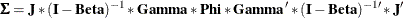whereis an identity matrix,is a selection matrix that contains 0 or 1 as its elements for selecting the covariance structures elements for the observed variables,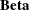is a square matrix for specifying relationships among the endogenous variables, Gamma is a matrix for specifying relationships between the endogenous variables and the exogenous variables, and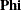is a matrix for specifying the variances and covariances of the exogenous variables. Notice that in the EQS model, error or disturbance variables are counted as exogenous variables in the model.

In Example 29.23, you use the following LINEQS specification for the Wheaton data:

proc calis nobs=932 data=Wheaton primat nose;
lineqs
Anomie67     = 1.0    * f_Alien67 + e1,
Powerless67  = 0.833  * f_Alien67 + e2,
Anomie71     = 1.0    * f_Alien71 + e3,
Powerless71  = 0.833  * f_Alien71 + e4,
Education    = 1.0    * f_SES     + e5,
SEI          = lambda * f_SES     + e6,
f_Alien67    = gamma1 * f_SES     + d1,
f_Alien71    = gamma2 * f_SES     + beta * f_Alien67 + d2;
variance
E1           = theta1,
E2           = theta2,
E3           = theta1,
E4           = theta2,
E5           = theta3,
E6           = theta4,
D1           = psi1,
D2           = psi2,
f_SES        = phi;
cov
E1  E3       = theta5,
E2  E4       = theta5;
run;


In the LINEQS statement, you specify all the functional relationships among variables. In the VARIANCE and COV statements, you specify all the variance and covariance parameters in the model. None of the parameters is specified as a matrix element in the LINEQS model. The default output by PROC CALIS does not print the EQS model matrices. To print these model matrices, you use the PRIMAT option in the PROC CALIS statement. Output 29.29.10, Output 29.29.11, and Output 29.29.12 show the initial specification of these model matrices:

Output 29.29.10: The Initial _EQSBETA_ Matrix by the LINEQS Model Specification

Initial _EQSBETA_ Matrix
Anomie67 Anomie71 Education Powerless67 Powerless71 SEI f_Alien67 f_Alien71
Anomie67
 0
 0
 0
 0
 0
 0
 1.0000
 0
Anomie71
 0
 0
 0
 0
 0
 0
 0
 1.0000
Education
 0
 0
 0
 0
 0
 0
 0
 0
Powerless67
 0
 0
 0
 0
 0
 0
 0.8330
 0
Powerless71
 0
 0
 0
 0
 0
 0
 0
 0.8330
SEI
 0
 0
 0
 0
 0
 0
 0
 0
f_Alien67
 0
 0
 0
 0
 0
 0
 0
 0
f_Alien71
 0
 0
 0
 0
 0
 0
 . [beta]
 0

Output 29.29.11: The Initial _EQSGAMMA_ Matrix by the LINEQS Model Specification

Initial _EQSGAMMA_ Matrix
f_SES e1 e3 e5 e2 e4 e6 d1 d2
Anomie67
 0
 1.0000
 0
 0
 0
 0
 0
 0
 0
Anomie71
 0
 0
 1.0000
 0
 0
 0
 0
 0
 0
Education
 1.0000
 0
 0
 1.0000
 0
 0
 0
 0
 0
Powerless67
 0
 0
 0
 0
 1.0000
 0
 0
 0
 0
Powerless71
 0
 0
 0
 0
 0
 1.0000
 0
 0
 0
SEI
 . [lambda]
 0
 0
 0
 0
 0
 1.0000
 0
 0
f_Alien67
 . [gamma1]
 0
 0
 0
 0
 0
 0
 1.0000
 0
f_Alien71
 . [gamma2]
 0
 0
 0
 0
 0
 0
 0
 1.0000

Output 29.29.12: The Initial _EQSPHI_ Matrix by the LINEQS Model Specification

Initial _EQSPHI_ Matrix
f_SES e1 e3 e5 e2 e4 e6 d1 d2
f_SES
 . [phi]
 0
 0
 0
 0
 0
 0
 0
 0
e1
 0
 . [theta1]
 . [theta5]
 0
 0
 0
 0
 0
 0
e3
 0
 . [theta5]
 . [theta1]
 0
 0
 0
 0
 0
 0
e5
 0
 0
 0
 . [theta3]
 0
 0
 0
 0
 0
e2
 0
 0
 0
 0
 . [theta2]
 . [theta5]
 0
 0
 0
e4
 0
 0
 0
 0
 . [theta5]
 . [theta2]
 0
 0
 0
e6
 0
 0
 0
 0
 0
 0
 . [theta4]
 0
 0
d1
 0
 0
 0
 0
 0
 0
 0
 . [psi1]
 0
d2
 0
 0
 0
 0
 0
 0
 0
 0
 . [psi2]

In the COSAN modeling language, you need to provide the three initial model matrices and the covariance structure formula for, which is shown in the following statements:

proc calis cov data=Wheaton nobs=932 nose;
cosan
var = Anomie67 Anomie71 Education Powerless67 Powerless71 SEI,
J(8, IDE) * Beta(8, GEN, IMI) * Gamma(9, GEN) * Phi(9, SYM);
matrix Beta
[1 4 8   , 7] = 1.0  0.833  beta,
[2 5     , 8] = 1.0  0.833 ;
matrix Gamma
[3 6 7 8 , 1] = 1.0  lambda gamma1 gamma2,
[1,2]         = 8 *  1.0;
matrix Phi
[1,1] = phi 2*theta1 theta3 2*theta2 theta4 psi1 psi2,
[3,2] = theta5 ,
[6,5] = theta5 ;
vnames J     = [Anomie67 Anomie71 Education Powerless67 Powerless71 SEI
f_Alien67 f_Alien71],
Beta  = J,
Gamma = [f_SES e1 e3 e5 e2 e4 e6 d1 d2],
Phi   = Gamma;
run;


In the PROC CALIS statement, you provide the data set in the DATA= option and the number of observations in the NOBS= option. You use the NOSE option to turn off the computation of the standard error estimates.

In the VAR= option of the COSAN statement, you provide the list of observed variables for the analysis. You arrange the observed variables in such a way that they are in the same order as in Output 29.29.10, Output 29.29.10, and Output 29.29.12. This is useful for comparing the results from the LINEQS and COSAN model specifications. After the specification of the observed variables, you specify the covariance structure model in the COSAN statement. Again, you only need to specify "half" of it. That is, the specification J(8,IDE)*Beta(8,GEN,IMI)*Gamma(9,GEN)*Phi(9,SYM) in the COSAN statement automatically expands towhich is the required covariance structures. Matrix properties and transformation types are defined in the arguments for the matrices.

Next, you use three matrix statements to specify the parameters in the matrix elements. The specifications here reflect exactly the initial specifications for the LINEQS model matrices as shown in Output 29.29.10, Output 29.29.10, and Output 29.29.12.

In the VNAMES statement, you specify the column variable names for the matrices. The column variable names of thematrix include all the observed variable names and the names of the intended endogenous latent factors f_Alien67 and f_Alien71. The column variable names for thematrix are the same as those for matrix. The column variables for the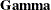matrix include the intended latent factor f_SES and error variable names e1e6 and d1d2, which are arranged in such a way that they match the order of the error variables in the LINEQS output shown in Output 29.29.12.

Output 29.29.13 shows the covariance structures and some properties of the model matrices. The covariance structure formula for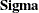is defined as required. You can also check the matrix properties in this output to see if they are what you intend them to be.

Output 29.29.13: The Covariance Structures and Model Matrices of the COSAN Model for the Wheaton Data: EQS Emulation

COSAN Model Structures
Sigma = J*inv(_I_-Beta)*Gamma*Phi*Gamma*(inv(_I_-Beta))*J

Summary of Model Matrices
Matrix N Row N Col Matrix Type
Beta 8 8 GEN: Square
Gamma 8 9 GEN: Rectangular
J 6 8 IDE: (I || 0)
Phi 9 9 SYM: Symmetric

Output 29.29.14 shows the model fit chi-square of the current COSAN model. As expected, this is the same model fit as in Output 29.17.7 of Example 29.17 and in Output 29.29.5.

Output 29.29.14: Model Fit of the COSAN Model for the Wheaton` Data: EQS Emulation

Fit Summary
Chi-Square 13.4851
Chi-Square DF 9
Pr > Chi-Square 0.1419

Output 29.29.15 shows the estimates of thematrix by the COSAN model specification. These estimates are essentially the same as the estimates of the _EQSBETA_ matrix obtained from the LINEQS model specification, as shown in Output 29.29.16.

Output 29.29.15: Estimate of theMatrix by the COSAN Model Specification

Model Matrix Beta
(8 x 8 General Square Matrix)
Anomie67 Anomie71 Education Powerless67 Powerless71 SEI f_Alien67 f_Alien71
Anomie67
 0
 0
 0
 0
 0
 0
 1.0000
 0
Anomie71
 0
 0
 0
 0
 0
 0
 0
 1.0000
Education
 0
 0
 0
 0
 0
 0
 0
 0
Powerless67
 0
 0
 0
 0
 0
 0
 0.8330
 0
Powerless71
 0
 0
 0
 0
 0
 0
 0
 0.8330
SEI
 0
 0
 0
 0
 0
 0
 0
 0
f_Alien67
 0
 0
 0
 0
 0
 0
 0
 0
f_Alien71
 0
 0
 0
 0
 0
 0
 0.5931 [beta]
 0

Output 29.29.16: Estimate of the _EQSBETA_ Matrix by the LINEQS Model Specification

_EQSBETA_ Matrix
Anomie67 Anomie71 Education Powerless67 Powerless71 SEI f_Alien67 f_Alien71
Anomie67
 0
 0
 0
 0
 0
 0
 1.0000
 0
Anomie71
 0
 0
 0
 0
 0
 0
 0
 1.0000
Education
 0
 0
 0
 0
 0
 0
 0
 0
Powerless67
 0
 0
 0
 0
 0
 0
 0.8330
 0
Powerless71
 0
 0
 0
 0
 0
 0
 0
 0.8330
SEI
 0
 0
 0
 0
 0
 0
 0
 0
f_Alien67
 0
 0
 0
 0
 0
 0
 0
 0
f_Alien71
 0
 0
 0
 0
 0
 0
 0.5931 [beta]
 0

Output 29.29.17 shows the estimates of thematrix by the COSAN model specification. Again, these estimates are essentially the same as the estimates of the _EQSGAMMA_ matrix obtained from the LINEQS model specification, as shown in Output 29.29.18.

Output 29.29.17: Estimate of the Gamma Matrix by the COSAN Model Specification

Model Matrix Gamma
(8 x 9 General Rectangular Matrix)
f_SES e1 e3 e5 e2 e4 e6 d1 d2
Anomie67
 0
 1.0000
 0
 0
 0
 0
 0
 0
 0
Anomie71
 0
 0
 1.0000
 0
 0
 0
 0
 0
 0
Education
 1.0000
 0
 0
 1.0000
 0
 0
 0
 0
 0
Powerless67
 0
 0
 0
 0
 1.0000
 0
 0
 0
 0
Powerless71
 0
 0
 0
 0
 0
 1.0000
 0
 0
 0
SEI
 5.3689 [lambda]
 0
 0
 0
 0
 0
 1.0000
 0
 0
f_Alien67
 -0.6299 [gamma1]
 0
 0
 0
 0
 0
 0
 1.0000
 0
f_Alien71
 -0.2409 [gamma2]
 0
 0
 0
 0
 0
 0
 0
 1.0000

Output 29.29.18: Estimate of the _EQSGAMMA_ Matrix by the LINEQS Model Specification

_EQSGAMMA_ Matrix
f_SES e1 e3 e5 e2 e4 e6 d1 d2
Anomie67
 0
 1.0000
 0
 0
 0
 0
 0
 0
 0
Anomie71
 0
 0
 1.0000
 0
 0
 0
 0
 0
 0
Education
 1.0000
 0
 0
 1.0000
 0
 0
 0
 0
 0
Powerless67
 0
 0
 0
 0
 1.0000
 0
 0
 0
 0
Powerless71
 0
 0
 0
 0
 0
 1.0000
 0
 0
 0
SEI
 5.3688 [lambda]
 0
 0
 0
 0
 0
 1.0000
 0
 0
f_Alien67
 -0.6299 [gamma1]
 0
 0
 0
 0
 0
 0
 1.0000
 0
f_Alien71
 -0.2409 [gamma2]
 0
 0
 0
 0
 0
 0
 0
 1.0000

Finally, Output 29.29.19 shows the estimates of thematrix by the COSAN model specification. These estimates are essentially the same as the estimates of the _EQSPHI_ matrix obtained from the LINEQS model specification, as shown in Output 29.29.20.

Output 29.29.19: Estimate of theMatrix by the COSAN Model Specification

Model Matrix Phi
(9 x 9 Symmetric Matrix)
f_SES e1 e3 e5 e2 e4 e6 d1 d2
f_SES
 6.6162 [phi]
 0
 0
 0
 0
 0
 0
 0
 0
e1
 0
 3.6078 [theta1]
 0.9058 [theta5]
 0
 0
 0
 0
 0
 0
e3
 0
 0.9058 [theta5]
 3.6078 [theta1]
 0
 0
 0
 0
 0
 0
e5
 0
 0
 0
 2.9938 [theta3]
 0
 0
 0
 0
 0
e2
 0
 0
 0
 0
 3.5950 [theta2]
 0.9058 [theta5]
 0
 0
 0
e4
 0
 0
 0
 0
 0.9058 [theta5]
 3.5950 [theta2]
 0
 0
 0
e6
 0
 0
 0
 0
 0
 0
 259.5738 [theta4]
 0
 0
d1
 0
 0
 0
 0
 0
 0
 0
 5.6705 [psi1]
 0
d2
 0
 0
 0
 0
 0
 0
 0
 0
 4.5148 [psi2]

Output 29.29.20: Estimate of the _EQSPHI_ Matrix by the LINEQS Model Specification

_EQSPHI_ Matrix
f_SES e1 e3 e5 e2 e4 e6 d1 d2
f_SES
 6.6163 [phi]
 0
 0
 0
 0
 0
 0
 0
 0
e1
 0
 3.6080 [theta1]
 0.9058 [theta5]
 0
 0
 0
 0
 0
 0
e3
 0
 0.9058 [theta5]
 3.6080 [theta1]
 0
 0
 0
 0
 0
 0
e5
 0
 0
 0
 2.9937 [theta3]
 0
 0
 0
 0
 0
e2
 0
 0
 0
 0
 3.5949 [theta2]
 0.9058 [theta5]
 0
 0
 0
e4
 0
 0
 0
 0
 0.9058 [theta5]
 3.5949 [theta2]
 0
 0
 0
e6
 0
 0
 0
 0
 0
 0
 259.5764 [theta4]
 0
 0
d1
 0
 0
 0
 0
 0
 0
 0
 5.6705 [psi1]
 0
d2
 0
 0
 0
 0
 0
 0
 0
 0
 4.5148 [psi2]